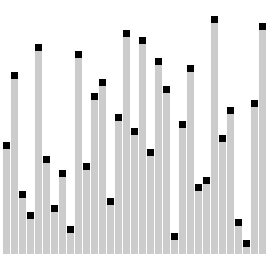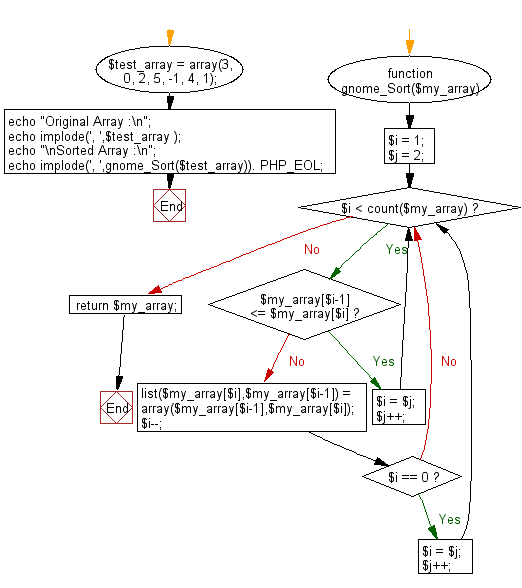# JavaScript Sorting Algorithm: Comb sort

## JavaScript Sorting Algorithm: Exercise-9 with Solution

Write a JavaScript program to sort a list of elements using Comb sort.

The Comb Sort is a variant of the Bubble Sort. Like the Shell sort, the Comb Sort increases the gap used in comparisons and exchanges. Some implementations use the insertion sort once the gap is less than a certain amount. The basic idea is to eliminate turtles, or small values near the end of the list, since in a bubble sort these slow the sorting down tremendously. Rabbits, large values around the beginning of the list, do not pose a problem in bubble sort.
In bubble sort, when any two elements are compared, they always have a gap of 1. The basic idea of comb sort is that the gap can be much more than 1.

Visualization of comb sort :Animation credits : Jerejesse

Sample Solution: -

HTML Code:

``````<!DOCTYPE html>
<html>
<meta charset="utf-8">
<title>JavaScript program of Comb sort</title>
<body></body>
</html>
```
```

JavaScript Code:

``````function combsort(arr)
{
function is_array_sorted(arr) {
var sorted = true;
for (var i = 0; i < arr.length - 1; i++) {
if (arr[i] > arr[i + 1]) {
sorted = false;
break;
}
}
return sorted;
}

var iteration_count = 0;
var gap = arr.length - 2;
var decrease_factor = 1.25;

// Repeat iterations Until array is not sorted

while (!is_array_sorted(arr))
{
// If not first gap  Calculate gap
if (iteration_count > 0)
gap = (gap == 1) ? gap : Math.floor(gap / decrease_factor);

// Set front and back elements and increment to a gap
var front = 0;
var back = gap;
while (back <= arr.length - 1)
{
// Swap the elements if they are not ordered

if (arr[front] > arr[back])
{
var temp = arr[front];
arr[front] = arr[back];
arr[back] = temp;
}

// Increment and re-run swapping

front += 1;
back += 1;
}
iteration_count += 1;
}
return arr;
}

var arra = [3, 0, 2, 5, -1, 4, 1];
console.log("Original Array Elements");
console.log(arra);
console.log("Sorted Array Elements");
console.log(combsort(arra));
```
```

Sample Output:

```Original Array Elements
[3,0,2,5,-1,4,1]
Sorted Array Elements
[-1,0,1,2,3,4,5]
```

Flowchart:Live Demo:

See the Pen searching-and-sorting-algorithm-exercise-9 by w3resource (@w3resource) on CodePen.

Improve this sample solution and post your code through Disqus

What is the difficulty level of this exercise?

Test your Programming skills with w3resource's quiz.

﻿